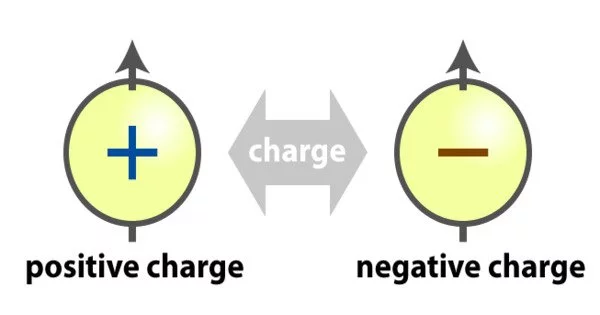Physics

# Electric ChargeWhen placed in an electromagnetic field, the physical property of matter known as electric charge causes matter to experience a force. Electric charge can be positive or negative (by convention, protons and electrons carry positive and negative charges). Like charges repel one another, while unlike charges attract one another. A neutral object is one that does not have any net charge. Classical electrodynamics refers to early knowledge of how charged substances interact, and it is still accurate for problems that do not require consideration of quantum effects.

A moving electric charge generates a magnetic field, which is associated with an electric field. The electromagnetic field is a combination of electric and magnetic fields. The interaction of the charges produces an electromagnetic force, which is the basis of physics.

Electric charge is quantized, which means it exists in discrete rather than continuous units. The elementary charge, denoted as “e,” is the fundamental unit of electric charge and is approximately equal to 1.602 x 10-19 coulombs (C). Charged particles can be positive or negative, with the magnitude of the charge determined by a whole number multiple of the elementary charge.

Types of Electric Charge

Two kinds of electric charges are there

• positive(+) charge
• negative(-) charge

Negative Charge – When an object has a negative charge it means that it has more electrons than protons.

Positive Charge – When an object has a positive charge it means that it has more protons than electrons.

When there is an identical number of positive and negative charges, the negative and positive charges would cancel out each other and the object would become neutral.

Electric charge is a conserved property; the net charge of an isolated system, which is the sum of positive and negative charges, cannot change. Subatomic particles carry electric charge. In ordinary matter, electrons carry negative charge, while protons carry positive charge in the nuclei of atoms. If there are more electrons than protons in a piece of matter, it has a negative charge, fewer have a positive charge, and equal numbers have a neutral charge.